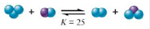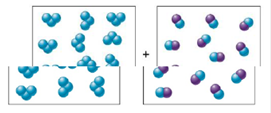Chapter 13, Problem 2ALQ

Chapter
Section
Textbook Problem

# The boxes shown below represent a set of initial conditions for the reaction:Draw a quantitative molecular picture that shows what this system looks like after the reactants are mixed in one of the boxes and the system reaches equilibrium. Support your answer with calculations.Interpretation Introduction

Interpretation: A set of initial conditions represented using boxes is given. A quantitative molecular picture that shows what this system will look like after the reactants are mixed in one of the boxes is to be obtained using calculations.

Concept introduction: When a reaction reaches the equilibrium state, the equilibrium ratio is expressed by the formula,

K=ConcentrationofproductsConcentrationofreactants

To determine: A quantitative molecular picture depicting what the given system will look like after the reaction reaches equilibrium.

Explanation

The given reaction is,

Figure 1

12 molecules of each reactant are given.

At equilibrium, the equilibrium ratio is expressed by the formula,

K=ConcentrationofproductsConcentrationofreactants

Where,

• K is the equilibrium constant.

The value of K is given to be 25 . Substitute this value in the above expression.

25=[NC][ND][NA][NB]

Where,

• N is the number of moles of each species involved.

The concentration of the products formed is assumed to be ‘ x ’ for each product.

The concentration of each reactant reduces by ‘ x ’.

Substitute this value in the above expression

### Still sussing out bartleby?

Check out a sample textbook solution.

See a sample solution

#### The Solution to Your Study Problems

Bartleby provides explanations to thousands of textbook problems written by our experts, many with advanced degrees!

Get Started